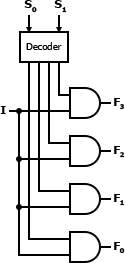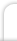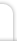Logic and Circuits
 Author: William Pantoja Illustrations: William Pantoja Date: December 22, 2011

Contents

1. Overview

2. Terminology

2.1. Negation

Negation, also called logical compliment, is an operation on one logical value that produces a value of true when the operand of the negation is false and a value of false when the operand of the negation is true.

2.2. Conjunction

Conjunction, also called logical conjunction, is an operation on two or more logical values that produces a value of true when all of the operands of the conjunction are true and a value of false when at least one of the operands of the conjunction is false.

2.3. Disjunction

Disjunction, also called logical disjunction, is an operation on two or more logical values that produces a value of true when at least one of the operands of the disjunction are true and a value of false when none of the operands of the disjunction are true.

2.4. Exclusive Disjunction

Exclusive disjunction, also called logical exclusive disjunction, is an operation on two or more logical values that produces a value of true when only one of the operands of the exclusive disjunction are true and a value of false when more than one the operands of the exclusive disjunction are true or when all of the operands of the exclusive disjunction are false.

3. Notation

3.1. TRUE

Notation Used In
T
1 Programming
Boolean algebra
TRUE Programming

3.2. FALSE

Notation Used In
F
0 Programming
Boolean algebra
FALSE Programming

3.3. A

Notation Used In
A

3.4. B

Notation Used In
B

3.5. NOT A

Notation Used In
!A Programming
~A Programming (bitwise)
NOT A Programming
¬ A Boolean algebra
A Boolean algebra
-A Programming (math), Mathematics
A

3.6. NOT B

Notation Used In
!B Programming
~B Programming (bitwise)
NOT B Programming
¬ B Boolean algebra
B Boolean algebra
-B Programming (math), Mathematics
B

3.7. A AND B

Notation Used In
A & B Programming (bitwise)
A && B Programming
A AND B Programming
AB Mathematics
AB Boolean algebra
AB Mathematics

3.8. A OR B

Notation Used In
A | B Programming (bitwise)
A || B Programming
A OR B Programming
A + B Mathematics
AB Boolean algebra

3.9. A XOR B

Notation Used In
A ^ B Programming, Programming (bitwise)
A XOR B Programming
AB Boolean algebra
AB Boolean algebra
AB

3.10. A NAND B

Notation Used In
AB Boolean algebra
A NAND B

3.11. A NOR B

Notation Used In
AB Boolean algebra
A NOR B

3.12. A XNOR B

Notation Used In
A XNOR B Programming
AB Boolean algebra
AB
A IFF B

4. Truth Tables

4.1. TRUE

INPUT OUTPUT
T T

4.2. FALSE

INPUT OUTPUT
F F

4.3. A

INPUT OUTPUT
A A
F F
T T

4.4. B

INPUT OUTPUT
B B
F F
T T

4.5. NOT A

INPUT OUTPUT
A NOT A
F T
T F

4.6. NOT B

INPUT OUTPUT
B NOT B
F T
T F

4.7. A AND B

INPUT OUTPUT
A B A AND B
F F F
F T F
T F F
T T T

4.8. A OR B

INPUT OUTPUT
A B A OR B
F F F
F T T
T F T
T T T

4.9. A XOR B

INPUT OUTPUT
A B A XOR B
F F F
F T T
T F T
T T F

4.10. A NAND B

INPUT OUTPUT
A B A NAND B
F F T
F T T
T F T
T T F

4.11. A NOR B

INPUT OUTPUT
A B A NOR B
F F T
F T F
T F F
T T F

4.12. A XNOR B

INPUT OUTPUT
A B A XNOR B
F F T
F T F
T F F
T T T

5. Venn Diagrams

The following Venn diagrams illustrate the basic logic operations. The inputs are two boolean values: A and B. The shaded areas represent where the output would produce a value of true. The white areas represent where the output would produce false.

5.1. TRUE

The output is always true.5.2. FALSE

The output is always false.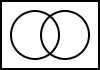5.3. A

The output is always the value of A.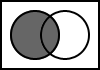5.4. B

The output is always the value of B.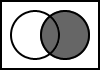5.5. NOT A

The output is the result of the negation of A.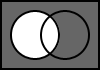5.6. NOT B

The output is the result of the negation of B.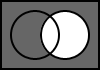5.7. A AND B

The output is the result of the logical conjunction of A and B.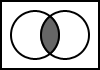5.8. A OR B

The output is the result of the logical disjunction of A and B.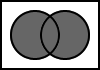5.9. A XOR B

The output is the result of the logical exclusive disjunction of A and B.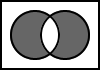5.10. A NAND B

The output is the result of the negation of the logical conjunction of A and B.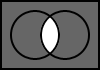5.11. A NOR B

The output is the result of the negation of the logical disjunction of A and B.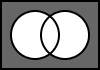5.12. A XNOR B

The output is the result of the negation of the logical exclusive disjunction of A and B.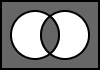6. Logic Gates

6.1. NOT

Gate StandardMIL/ANSI/IEEE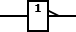IEC

6.2. AND

Gate StandardMIL/ANSI/IEEE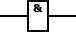IEC

6.3. OR

Gate Standard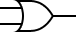MIL/ANSI/IEEEIEC

6.4. XOR

Gate Standard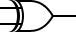MIL/ANSI/IEEE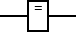IEC

6.5. NAND

Gate StandardMIL/ANSI/IEEE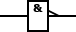IEC

6.6. NOR

Gate Standard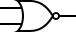MIL/ANSI/IEEE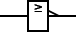IEC

6.7. XNOR

Gate Standard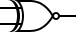MIL/ANSI/IEEE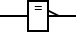IEC

7. Boolean Algebra

7.1. Associativity

Within an expression containing two or more occurrences of the same associative operator, the order in which the operations are performed does not matter as long as the sequence of the operands is not changed.

A ∧ (BC) ≡ (AB) ∧ C
A ∨ (BC) ≡ (AB) ∨ C

7.2. Commutativity

Changing the order of the operands does not change the end result.

ABBA
ABBA

7.3. Distributivity

The value on the left side of the operation may be distributed within the expression on the right side of the operation.

A ∧ (BC) ≡ (AB) ∨ AC
A ∨ (BC) ≡ (AB) ∧ AC

7.4. Identity

The result of the operation is equivalent to value on the left side of the operation.

A ∧ 1 ≡ A
A ∨ 0 ≡ A

7.5. Annihilator

The value on the left side of the operation is annihilated.

A ∧ 0 ≡ 0
A ∨ 1 ≡ 1

7.6. Idempotence

The operation has no effect on the value of the left side of the operation.

AAA
AAA

7.7. Absorbtion

The expression on the right side of the operation is absorbed.

A ∧ (AB) ≡ A
A ∨ (AB) ≡ A

7.8. Complementation

The expression always yields a value of true or false.

A ∧ ¬A ≡ 0
A ∨ ¬A ≡ 1

7.9. Double Negation

A double negation of a value is equivalent to the value.

¬¬AA

7.10. De Morgan's Laws

The negation of a conjunction is the disjunction of the negations and the negation of a disjunction is the conjunction of the negations.

AB ≡ ¬(¬A ∨ ¬B)
AB ≡ ¬(¬A ∧ ¬B)
¬(AB) ≡ ¬A ∨ ¬B
¬(AB) ≡ ¬A ∧ ¬B

8. Circuits

8.1. Universal Gates

Universal logic gates are called that because they can be used to create every other type of logic gate. The two logic gates NAND and NOR are universal logic gates. NAND gates are the most commonly used because they require fewer gates than NOR to create the other logic gates.

8.1.1. NOR

Gate Logic Circuit
NOT ¬AAA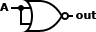AND AB ≡ (AA) ↓ (BB)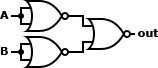OR AB ≡ (AB) ↓ (AB)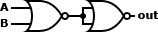XOR AB ≡ ((AA) ↓ (BB)) ↓ (AB)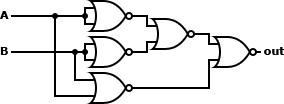NAND AB ≡ ((AA) ↓ (BB)) ↓ ((AA) ↓ (BB))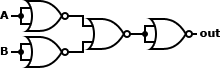NOR ABAB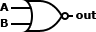XNOR AB ≡ (((AA) ↓ (BB)) ↓ (AB)) ↓ (((AA) ↓ (BB)) ↓ (AB))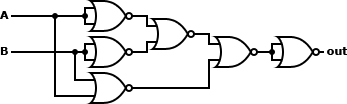8.1.2. NAND

Gate Logic Circuit
NOT ¬AAA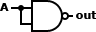AND AB ≡ (AB) ↑ (AB)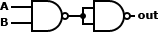OR AB ≡ (AA) ↑ (BB)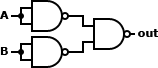XOR AB ≡ (A ↑ (AB)) ↑ (B ↑ (AB))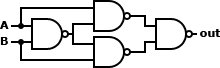NAND ABAB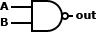NOR AB ≡ ((AA) ↑ (BB)) &uarr ((AA) ↑ (BB))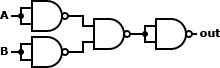XNOR AB ≡ ((A ↑ (AB)) ↑ (B ↑ (AB))) ↑ ((A ↑ (AB)) ↑ (B ↑ (AB)))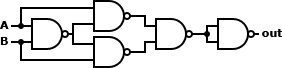8.2. Complex Circuits

A half adder adds two one-bit binary numbers, A and B. The outputs are S and C (the value theoretically carried over to the next addition operation). The final summ is 2S + C. The half adder cannot be chained together because of the inability to use the carry from the last operation as an input.

Truth Table:

INPUT OUTPUT
A B S C
F F F F
F T T F
T F T F
T T F T

Circuit:Example: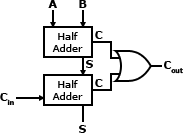A full adder operates in the same way a half adder does. However, because the full adder takes a carry value (Cin) as input a full adder may be chained together to create an adder for a larger number.

Truth Table:

INPUT OUTPUT
A B Cin S Cout
F F F F F
F F T T F
F T F T F
F T T F T
T F F T F
T F T F T
T T F F T
T T T T T

Circuit: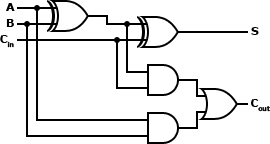Example: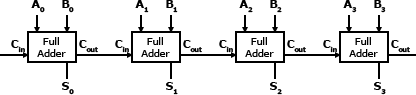8.2.3. Decoder

A decoder reverses the operation of an encoder. The original data is reconstituted from the encoded data into its original form. A decoder is commonly used to create a multiplexer and demultiplexer.

Truth Table:

INPUT OUTPUT
S0 S1 D3 D2 D1 D0
F F F F F T
F T F F T F
T F F T F F
T T T F F F

Circuit: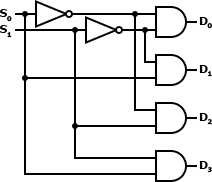8.2.4. Multiplexer

A multiplexer is used to combine multiple streams of data into a single stream. The resultant data stream is split back into its original data streams using a demultiplexer.

Truth Table:

INPUT OUTPUT
S1 S0 I3 I2 I1 I0 F
F F F F F F F
F F F F F T T
F F F F T F F
F F F F T T T
F F F T F F F
F F F T F T T
F F F T T F F
F F F T T T T
F F T F F F F
F F T F F T T
F F T F T F F
F F T F T T T
F F T T F F F
F F T T F T T
F F T T T F F
F F T T T T T
F T F F F F F
F T F F F T F
F T F F T F T
F T F F T T T
F T F T F F F
F T F T F T F
F T F T T F T
F T F T T T T
F T T F F F F
F T T F F T F
F T T F T F T
F T T F T T T
F T T T F F F
F T T T F T F
F T T T T F T
F T T T T T T
T F F F F F F
T F F F F T F
T F F F T F F
T F F F T T F
T F F T F F T
T F F T F T T
T F F T T F T
T F F T T T T
T F T F F F F
T F T F F T F
T F T F T F F
T F T F T T F
T F T T F F T
T F T T F T T
T F T T T F T
T F T T T T T
T T F F F F F
T T F F F T F
T T F F T F F
T T F F T T F
T T F T F F F
T T F T F T F
T T F T T F F
T T F T T T F
T T T F F F T
T T T F F T T
T T T F T F T
T T T F T T T
T T T T F F T
T T T T F T T
T T T T T F T
T T T T T T T

Circuit:8.2.5. Demultiplexer

A demultiplexer splits a data stream that was combined using a multiplexer back into its original composite data streams.

Truth Table:

INPUT OUTPUT
S1 S0 I F3 F2 F1 F0
F F F F F F F
F F T F F F T
F T F F F F F
F T T F F T F
T F F F F F F
T F T F T F F
T T F F F F F
T T T T F F F

Circuit: